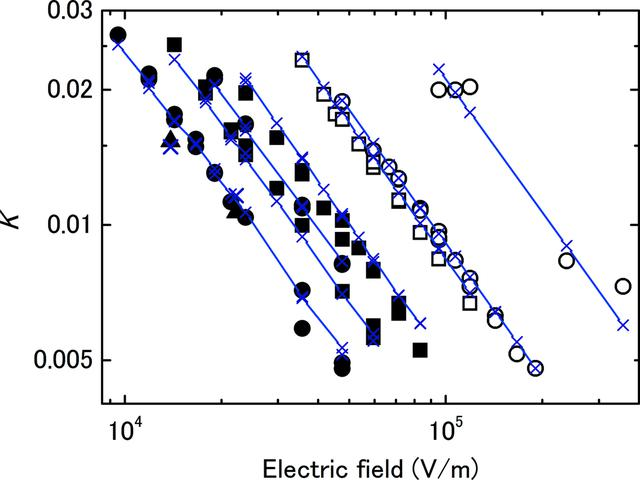disable zoom     view article Figure 5 Plots of K against E demonstrating that K is inversely proportional to E. The circles, squares and triangles denote IC1, IC2 and IC3, respectively. The open and closed marks are data for 10 and 15 keV X-rays, respectively. The lines with crosses denote the calculated K values using equation (7), in which the value of 3.02 × 1010 V m C−1 was used for μ (ICRU, 1982). The charge per pulse ranged from 0.4 to 1.7 pC.JOURNAL OFSYNCHROTRONRADIATION
ISSN: 1600-5775
Volume 20| Part 5| July 2013| Pages 698-704